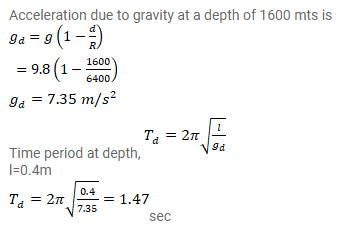# A simple pendulum of length 40cm is taken inside a deep mine.

Question:

A simple pendulum of length $40 \mathrm{~cm}$ is taken inside a deep mine. Assume for the time being that the mine is $1600 \mathrm{~km}$ deep. Calculate the time period of the pendulum there. Radius of the earth $=6400 \mathrm{~km}$.

Solution: